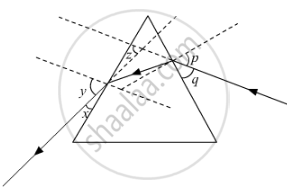Share

# In this diagram, the angle of incidence, the angle of emergence and the angle of deviation respectively have been represented by - CBSE Class 10 - Science

#### Question

Study the following ray diagram:In this diagram, the angle of incidence, the angle of emergence and the angle of deviation respectively have been represented by

(A) y, p, z

(B) x, q, z

(C) p, y, z

(D) p, z, y

#### Solution

Angle p is the angle of incidence since this angle is formed between the incident ray and the normal.

Angle y is the angle of emergence as it is formed between the emergent ray and the normal.

and angle z is the angle of deviation as it is formed between the emergent ray and the incident ray.

Hence, the correct answer is p, y, z

Is there an error in this question or solution?
Solution In this diagram, the angle of incidence, the angle of emergence and the angle of deviation respectively have been represented by Concept: Dispersion by a Prism.
S